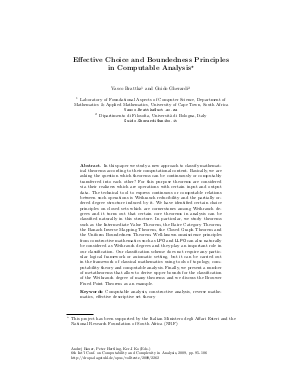Document# Effective Choice and Boundedness Principles in Computable Analysis

### Authors Vasco Brattka, Guido Gherardi## File

OASIcs.CCA.2009.2262.pdf
• Filesize: 379 kB
• 12 pages

## Cite As

Vasco Brattka and Guido Gherardi. Effective Choice and Boundedness Principles in Computable Analysis. In 6th International Conference on Computability and Complexity in Analysis (CCA'09). Open Access Series in Informatics (OASIcs), Volume 11, pp. 95-106, Schloss Dagstuhl - Leibniz-Zentrum für Informatik (2009)
https://doi.org/10.4230/OASIcs.CCA.2009.2262

## Abstract

In this paper we study a new approach to classify mathematical theorems according to their computational content. Basically, we are asking the question which theorems can be continuously or computably transferred into each other? For this purpose theorems are considered via their realizers which are operations with certain input and output data. The technical tool to express continuous or computable relations between such operations is Weihrauch reducibility and the partially ordered degree structure induced by it. We have identified certain choice principles on closed sets which are cornerstones among Weihrauch degrees and it turns out that certain core theorems in analysis can be classified naturally in this structure. In particular, we study theorems such as the Intermediate Value Theorem, the Baire Category Theorem, the Banach Inverse Mapping Theorem, the Closed Graph Theorem and the Uniform Boundedness Theorem. Well-known omniscience principles from constructive mathematics such as \$\LPO\$ and \$\LLPO\$ can naturally be considered as Weihrauch degrees and they play an important role in our classification. Our classification scheme does not require any particular logical framework or axiomatic setting, but it can be carried out in the framework of classical mathematics using tools of topology, computability theory and computable analysis. Finally, we present a number of metatheorems that allow to derive upper bounds for the classification of the Weihrauch degree of many theorems and we discuss the Brouwer Fixed Point Theorem as an example.
##### Keywords
• Computable analysis
• constructive analysis
• reverse mathematics
• effective descriptive set theory

## Metrics

• Access Statistics
• Total Accesses (updated on a weekly basis)
0